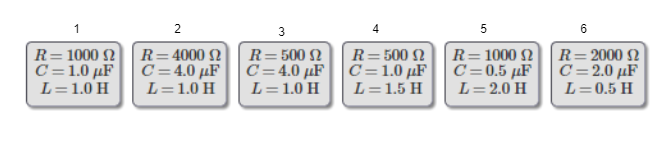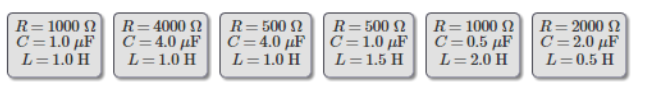# Problem: Each circuit is driven at its resonance frequency by a 100 Vrms AC power supply. Rank these circuits on the basis of their maximum current. Rank from largest to smallest. To rank items as equivalent, overlap them.

###### FREE Expert Solution

Maximum current:

$\overline{){{\mathbf{i}}}_{\mathbf{m}\mathbf{a}\mathbf{x}}{\mathbf{=}}\frac{\mathbf{V}}{\mathbf{R}}}$

Label the circuits as follows:88% (172 ratings)###### Problem Details

Each circuit is driven at its resonance frequency by a 100 Vrms AC power supply. Rank these circuits on the basis of their maximum current. Rank from largest to smallest. To rank items as equivalent, overlap them.